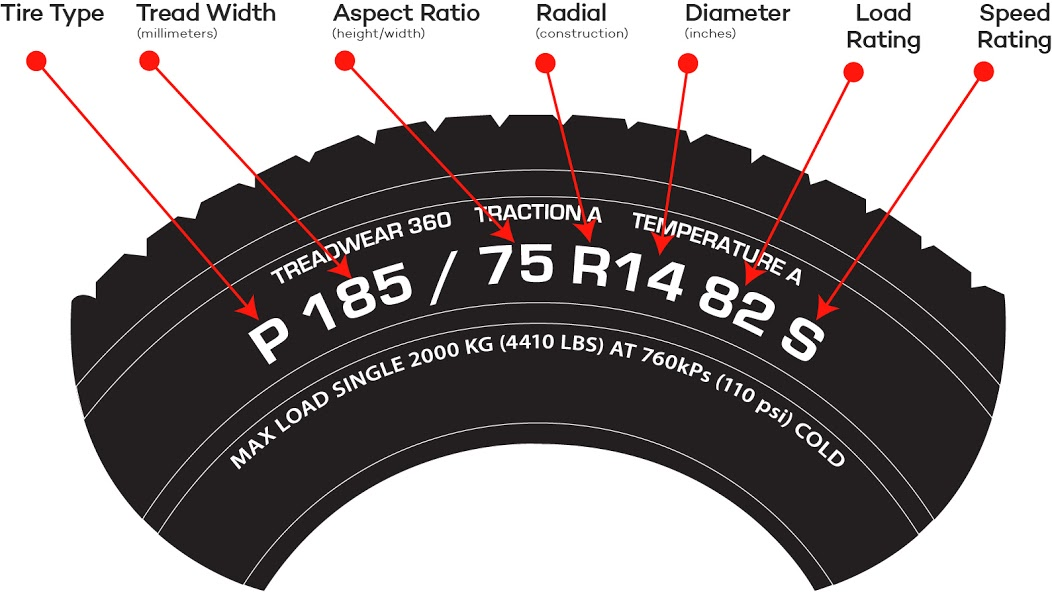## HOW DO I READ MY TIRE SPECS?

Formula for calculating tire dimensions;

### USING SIZE 185/75-14 FOR EXAMPLE

The dimensions of a  235/35-19 tire are 9.3” wide (section width) & 25.5” tall (overall dia) The section width is 235mm wide (There are 25.4mm to an inch)

#### STEP 1)

185mm divided by 25.4” = 7.28” (section width)

#### STEP 2)

Multiply 7.28 (section width) by .75 (aspect ratio)

7.28 x .75 = 5.46” (section height)

To find overall dia

Multiply 5.46 (section height) x 2  then add rim dia

#### STEP 3)

5.46” x 2 = 10.92” + 14.0” = 24.92” round out to 24.9”  (overall dia)